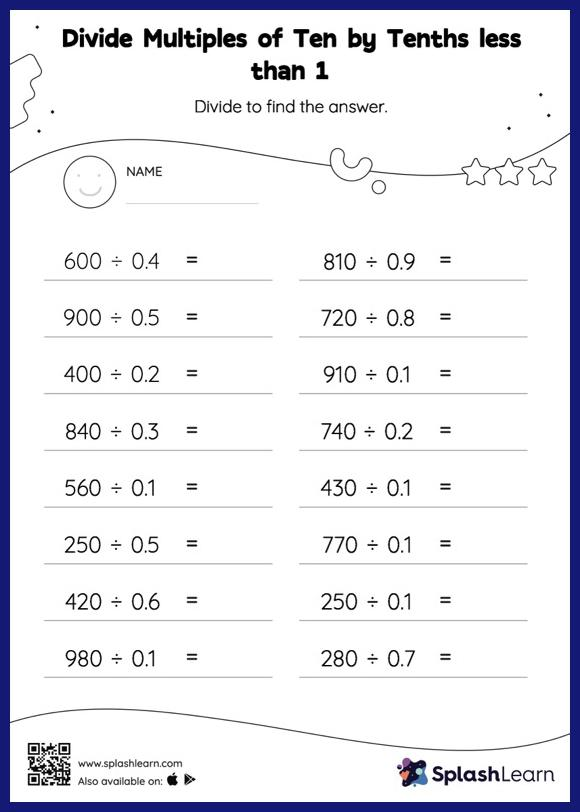# Divide Multiples of Ten by Tenths less than 1 without Remainder: Horizontal Division Worksheet

Home > Divide Multiples of Ten by Tenths less than 1 without Remainder: Horizontal DivisionWhen dividing a number by a decimal, students divide without considering the decimal point and then move each digit of the quotient to the left based on the number of decimal digits in the divisor. Divide multiples of ten by tenths less than 1 without remainder provides plenty of practice with this concept. As the worksheet uses a horizontal format, it allows for more creativity when it comes to how the student solves the problem. In contrast, in the vertical format, students usually employ the standard method to solve.